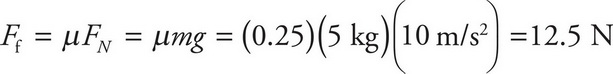# AP Physics 1 Question 385: Answer and Explanation

### Test Information

Question: 385

3. A box with a mass of 5 kg is sliding across a table at a speed of 2 m/s. The coefficient of kinetic friction between the book and table is μ = 0.25. What is the minimum force that has to be applied on the box to maintain this speed?

• A. 0 N
• B. 10 N
• C. 12.5 N
• D. 25 N